# MCAT Physical : Types of Image

## Example Questions

### Example Question #1 : Types Of Image

A man stands ten meters away from a converging mirror with a focal length of two meters. What is true of the image he sees?

His image is virtual, upright, and minimized

His image is real, inverted, and magnified

His image is real, inverted, and minimized

His image is real, upright, and magnified

His image is virtual, upright, and magnified

His image is real, inverted, and minimized

Explanation:

The first thing to consider when answering this question is the fact that real images are always inverted and virtual images are always upright. Once you have determined one or the other, two answer choices can be eliminated.

The first equation that is necessary for this question is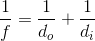.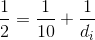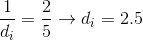From this we can determine that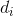is equal to. Sinceis a positive number we know the image is real, and thus inverted.

The second equation to consider is for magnification:.

If the absolute value ofis greater than one, the image is magnified, and if the value is less than one, it is minimized.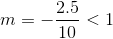We would expect the image to be minimized.

### Example Question #2 : Types Of Image

For a nearsighted person, the image of a distant object is formed __________.

behind the retina, and corrected using a converging lens

behind the retina, and corrected using a diverging lens

in front of the retina, and corrected using a converging lens

in front of the retina, and corrected using a diverging lens

in front of the retina, and corrected using a diverging lens

Explanation:

In nearsightedness, the person cannot see far objects due to increased refraction. This causes the image to be formed in front of the retina. This condition is corrected using a diverging lens to compensate for the "over refraction" by the deformed cornea.

### Example Question #3 : Types Of Image

An object is placed 50cm in front of a converging lens whose focal length is 20cm. Which of the following best describes the image that is formed?

The same size as the object and virtual

Smaller than the object and real

Larger than the object and real

Smaller than the object and virtual

Larger than the object and virtual

Smaller than the object and real

Explanation:

Relevant equations: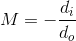Step 1: Plug in the given focal length and object distance to find the image distance: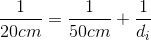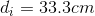Step 2: Find the magnification and orientation of the image: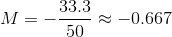A negative magnification means the image is inverted, and therefore real. A magnification smaller than 1 means the image is smaller than the object.

The following result is true, in general, for converging lenses: if the object is outside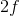, then the image is inverted, real, and smaller than the object.